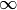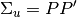# statsmodels.tsa.vector_ar.var_model.VARProcess¶

class statsmodels.tsa.vector_ar.var_model.VARProcess(coefs, intercept, sigma_u, names=None)[source]

Class represents a known VAR(p) process

Parameters: coefs : ndarray (p x k x k) intercept : ndarray (length k) sigma_u : ndarray (k x k) names : sequence (length k) Attributes:

Methods

 acf([nlags]) Compute theoretical autocovariance function acorr([nlags]) Compute theoretical autocorrelation function forecast(y, steps) Produce linear minimum MSE forecasts for desired number of steps forecast_cov(steps) Compute theoretical forecast error variance matrices forecast_interval(y, steps[, alpha]) Construct forecast interval estimates assuming the y are Gaussian get_eq_index(name) Return integer position of requested equation name is_stable([verbose]) Determine stability based on model coefficients long_run_effects() Compute long-run effect of unit impulse ma_rep([maxn]) Compute MA() coefficient matrices mean() Mean of stable process mse(steps) Compute theoretical forecast error variance matrices orth_ma_rep([maxn, P]) Compute Orthogonalized MA coefficient matrices using P matrix such that. plot_acorr([nlags, linewidth]) Plot theoretical autocorrelation function plotsim([steps]) Plot a simulation from the VAR(p) process for the desired number of